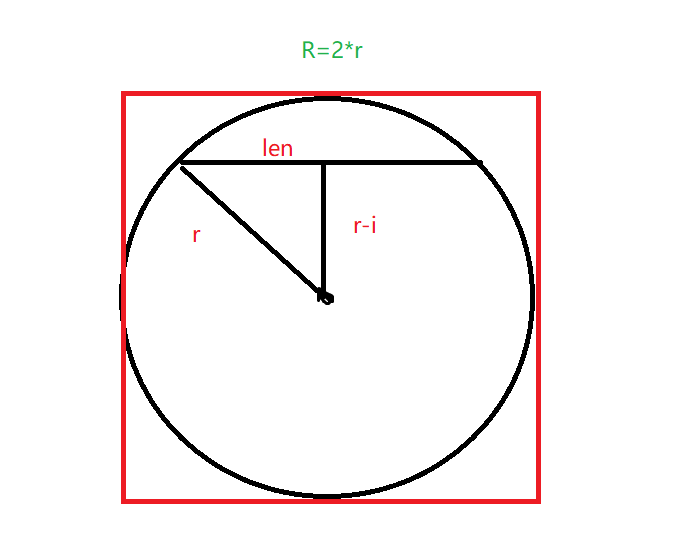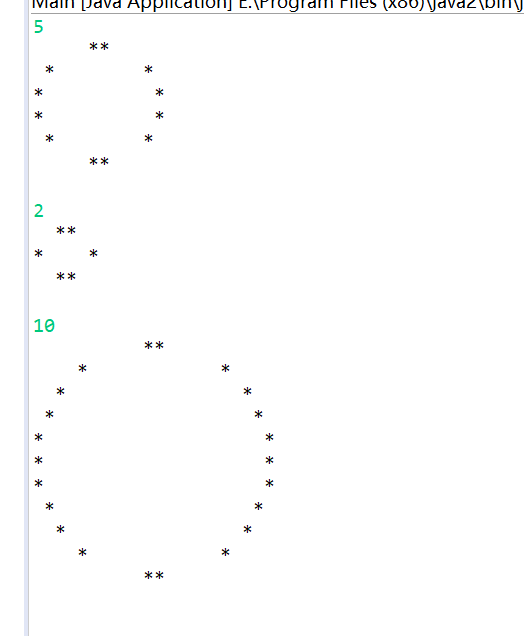# JAVA用“*”打印出圆形实验案例

• 首先如果每行都进行如上判断，那么会发现输出的圆成了“椭圆”，其高度差不多是宽度的两倍，故我们改变一下，每两行进行一次如上判断，可以输出一个近似圆。
• 其中几个函数作用如下：

Math.abs();//求绝对值
Math.sqrt();//求开方根
Math.round();//求近似整数public class Circle {
private double r;
double eps = 1;
Circle(double r){
this.r = r;
}
public void printCricle() {
double R = 2*r;
for(int i = 0;i <= R;i+=2) {
double y;
if(i <= r)	y = r-i;
else y = i-r;
double len =  Math.round(Math.sqrt(r*r - y*y));
for(int j = 0;j < r-len;j++)	System.out.print(" ");
System.out.print("*");
for(int j = 0;j < 2*len;j++)	System.out.print(" ");
System.out.println("*");
}
}
}©️2019 CSDN 皮肤主题: 终极编程指南 设计师: CSDN官方博客